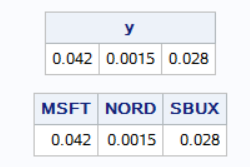## how do I preserve column names when returning a matrix from a function

The following little program assigns column names to a row vector mu_vec.  However, if this processing is carried out within a function, then the command "return(mu_vec);" will get rid of the column names.  Is there a way to preserve these column names?

``````assetNames={"MSFT" "NORD" "SBUX"};
mu_vec={0.042 0.0015 0.028};
mattrib mu_vec c=assetNames;
print mu_vec;``````

1 ACCEPTED SOLUTION

Accepted Solutions

## Re: how do I preserve column names when returning a matrix from a function

I assume that you are trying something like the following:

``````start Func(x);
assetNames={"MSFT" "NORD" "SBUX"};
mu_vec={0.042 0.0015 0.028};
mattrib mu_vec c=assetNames;
return mu_vec;
finish;

y = Func(x);  /* copies values but not atrtributes */
print y;``````

This doesn't work the way you hoped because inside the FUNC module, the variable X is a local variable. Any attributes you assign as assigned to the local variable and are not copied to the variable Y, which has a different scope. (See this article to learn more about the scope of variables in the SAS/IML language.)

If you want to give Y attributes, you need to assign them in the scope for Y. If the column names are known inside the function, you can return them (as part of a list) from the function. You can then call the MATTRIB statement in the parent environment. like this:

``````start Func2(x);
assetNames={"MSFT" "NORD" "SBUX"};
mu_vec={0.042 0.0015 0.028};
return ( [mu_vec, assetNames] );  /* return a list */
finish;

L = Func2(x);      /* output is a list with two items */
y = L\$1;           /* values are the first item in list */
mattrib y c=(L\$2); /* column names are the second item */
print y;
``````
6 REPLIES 6

## Re: how do I preserve column names when returning a matrix from a function

I assume that you are trying something like the following:

``````start Func(x);
assetNames={"MSFT" "NORD" "SBUX"};
mu_vec={0.042 0.0015 0.028};
mattrib mu_vec c=assetNames;
return mu_vec;
finish;

y = Func(x);  /* copies values but not atrtributes */
print y;``````

This doesn't work the way you hoped because inside the FUNC module, the variable X is a local variable. Any attributes you assign as assigned to the local variable and are not copied to the variable Y, which has a different scope. (See this article to learn more about the scope of variables in the SAS/IML language.)

If you want to give Y attributes, you need to assign them in the scope for Y. If the column names are known inside the function, you can return them (as part of a list) from the function. You can then call the MATTRIB statement in the parent environment. like this:

``````start Func2(x);
assetNames={"MSFT" "NORD" "SBUX"};
mu_vec={0.042 0.0015 0.028};
return ( [mu_vec, assetNames] );  /* return a list */
finish;

L = Func2(x);      /* output is a list with two items */
y = L\$1;           /* values are the first item in list */
mattrib y c=(L\$2); /* column names are the second item */
print y;
``````

## Re: how do I preserve column names when returning a matrix from a function

I love this solution. I didn't know about the list feature.
Thanks a lot.

## Re: how do I preserve column names when returning a matrix from a function

For an introduction to lists, see "Lists: Nonmatrix data structures in SAS/IML"

For an introduction to the list notational syntax, see "Create lists by using a natural syntax in SAS/IML"Ksharp
Super User

## Re: how do I preserve column names when returning a matrix from a function

Dr. Rick has said very clear.

Or could pass local matrix into global matrix ,if your IML is low version.

``````proc iml;

start Func(x) global(mu ,asset );
assetNames={"MSFT" "NORD" "SBUX"};
mu_vec={0.042 0.0015 0.028};
mattrib mu_vec c=assetNames;
mu=mu_vec ;asset=assetNames;
return mu_vec;
finish;

y = Func(x);  /* copies values but not atrtributes */
mattrib mu c=asset l='';
print y , mu ;

quit;``````## Re: how do I preserve column names when returning a matrix from a function

Thanks for your suggestion. Would global passing of a matrix overwrite a matrix of the same name in the parent program?Ksharp
Super User

## Re: how do I preserve column names when returning a matrix from a function

Yes. It is global. It would be overwrited if there are same name in parent program.
But you could define a unique name to avoid it .
From The DO Loop